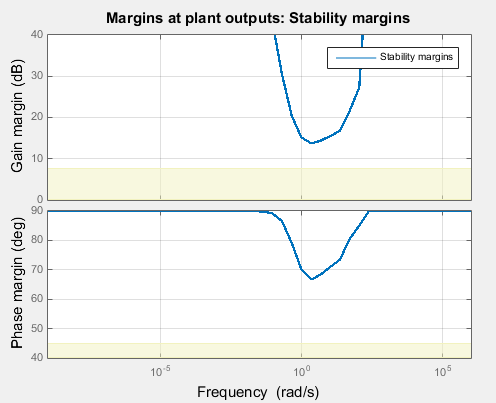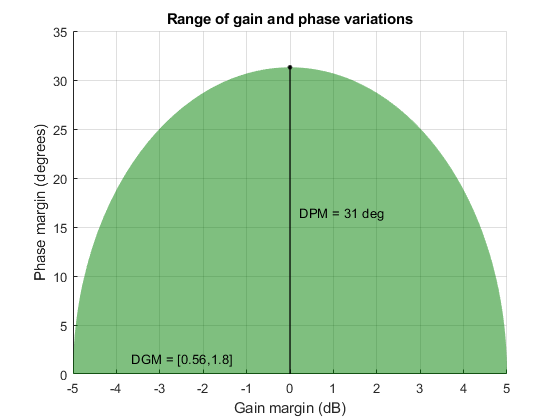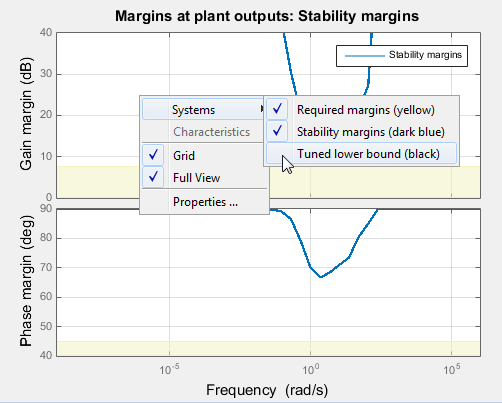## Stability Margins in Control System Tuning

In control system tuning, you specify target gain and phase margins using Margins Goal (for Control System Tuner) or `TuningGoal.Margins` (for `systune`). The software provides tools to help you visualize and interpret the gain and phase margins in your tuned system.

### Gain and Phase Margins

Gain and phase margins measure the tolerance of a control loop to variations in the open-loop system response. The Margins Goal and `TuningGoal.Margins` rely on the notion of a disk margin to compute gain and phase margins. Like classical gain and phase margins, disk margins quantify the stability of a closed-loop system against gain or phase variations in the open-loop response. Disk margins also take into account all frequencies and loop interactions. Therefore, disk-based margin analysis provides a stronger guarantee of stability than the classical gain and phase margins. For more information about disk margins, see Stability Analysis Using Disk Margins (Robust Control Toolbox).

For a SISO system, the gain and phase margins indicate how much the gain or phase of the open-loop response L can change without loss of stability.

For MIMO systems, gain and phase margins are interpreted as follows:

• Gain margin — Stability is preserved when the gain changes up to the gain margin value in each feedback channel. The gain can change in all channels simultaneously, and by a different amount in each channel.

• Phase margin — Stability is preserved when the phase changes up to the phase margin value in each feedback channel. The phase can change in all channels simultaneously, and by a different amount in each channel.

Gain and phase margins typically vary across frequencies. For example, in a SISO loop, a gain margin of 5 dB at 2 rad/s indicates that closed-loop stability is maintained when the loop gain increases or decreases by as much as 5 dB at this frequency. For control system tuning, you specify target values for the minimum (worst) margins across all frequencies. The margin tuning goal assumes symmetric ranges of variation, such as ±5 dB or ±30°.

### Interpret Gain and Phase Margin Plots

For control system tuning, visualize system stability margins to help evaluate the performance of the tuned system.

• In Control System Tuner, use a Margins Goal or Quick Loop Tuning.

• At the command line, use `viewGoal`. For instance, if `S` is the control system, and `Req` is a `TuningGoal.Margins` goal, enter the following.

`viewGoal(Req,S)`

`viewGoal` produces a plot with a yellow shaded region where the target margins are not met. The plot also shows the gain and phase margins for the current values of the tunable parameters in the control system. These margins appear as a blue trace that typically varies across frequencies. For instance, the following plot shows a typical result.The plot shows that the frequency of the gain or phase variation can affect how large a perturbation the system can tolerate without going unstable. The minimum (worst) gain and phase margins occur at about 2 rad/s. At this frequency, the system can tolerate changes in open-loop gain of about ±14 dB, or changes in phase of about ±66°. For this system, the margins at all frequencies are well above the target margins used for tuning, shown in yellow.

### Simultaneous Gain and Phase Variations

In general, gain margins are determined assuming no phase variation, and phase margins are determined assuming no gain variation. In practice, your system can experience simultaneous gain and phase variations. Disk-based margin analysis also gives you a range of simultaneous gain and phase variations that the system can tolerate. For instance, suppose that your system has a disk-based gain margin of 5 dB. This system remains stable for gain changes of ±5 dB, assuming no phase variation. Use the `diskmarginplot` (Robust Control Toolbox) command to visualize the region of simultaneous gain and phase variations that the system can tolerate.

`diskmarginplot(db2mag(5))`The shaded region shows the stable range of combined gain and phase variations for a disk-based gain margin of 5 dB. With no phase variation, the system can tolerate the full range of gain variation, –5 dB to 5 dB, or gain that changes by a factor within the range `DGM = [0.56,1.8]`. Adding in phase variation reduces the tolerable gain variation. For instance, If the phase is allowed to vary by ±25°, the tolerable gain variation drops to a range of about ±3 dB. The disk-based phase margin is the allowable phase variation when there is no gain variation, in this case about ±31°, shown in the plot as `DPM`.

### Algorithm

The gain and phase margin values are both derived from the disk margin. The disk margin measures the radius of a circular exclusion region centered near the critical point. (See Stability Analysis Using Disk Margins (Robust Control Toolbox).) For a system with open-loop response L(), this radius ɑ is a function of the scaled norm:

`$\frac{1}{\alpha }=\underset{D\text{\hspace{0.17em}}\text{diagonal}}{\mathrm{min}}{‖D{\left(j\omega \right)}^{-1}\left(I-L\left(j\omega \right)\right){\left(I+L\left(j\omega \right)\right)}^{-1}D\left(j\omega \right)‖}_{\infty }.$`

Unlike classical gain and phase margins, the disk margins and associated gain and phase margins guarantee that the open-loop response stays at a safe distance from the critical point at all frequencies.

#### Impact of Scaling

The frequency dependence of the gain and phase margins can be obtained by an exact calculation involving μ-analysis. However, for computational efficiency, the tuning algorithm uses an approximate calculation with a constant scaling D instead of the frequency-dependent scaling D():

`$\frac{1}{\alpha }=\underset{D\text{\hspace{0.17em}}\text{diagonal}}{\mathrm{min}}{‖{D}^{-1}\left(I-L\left(j\omega \right)\right){\left(I+L\left(j\omega \right)\right)}^{-1}D‖}_{\infty }.$`

This approximation is an upper bound on 1/ɑ, or a lower bound on ɑ. It can therefore yield smaller margins in parts of the frequency range, especially at frequencies away from the frequency at which the minimum margin occurs. The smaller margin is still a guaranteed margin, but it might be more conservative than the true margin. To see the lower bound used by the tuning algorithm, right-click on the stability-margins plot and select Systems > Tuned lower bound.If you see a significant gap between the actual margins of the tuned system (blue curve) and the lower-bound approximation used for tuning (black curve), try increasing the D-scaling order to introduce some frequency dependence into the scaling. For tuning in Control System Tuner, set the D-scaling order in the Margins Goal dialog box. For command-line tuning, set this value using the `ScalingOrder` property of `TuningGoal.Margins`. The default order is zero (static scaling).